# SOLVEDMoData Effector Rotation

Hello

I'm working on a plugin that takes in points from polygon objects and then uses effectors fed in by the user to change the positions of the points that I then draw.

With the code below I am able to change the positions of the points but the final result is different than the equivalent setup with a Matrix object as seen here. Both my code and the matrix are working from points set as the center point of the polygons of a Sphere with only a default Plain Effector in the Effector List.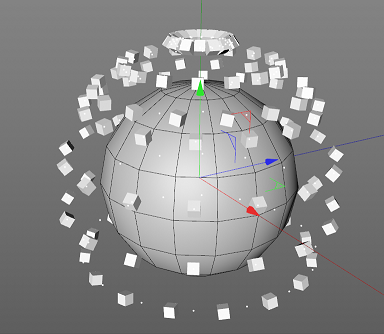My results are shown with the white dots compared to the cubes from the Matrix object.

``````
PolygonObject *poly = (PolygonObject*)doc->SearchObject("Sphere"_s);
if (!poly )
return;

maxon::BaseArray<Vector> drawPoints;
if (poly == nullptr)
return;

polygonMatrix = poly->GetMg();

const CPolygon *polyArray = poly->GetPolygonR();

if (polyArray == nullptr)
return;

Matrix objMx = poly->GetMg();

BaseObject *obj = (BaseObject*)Get();
BaseTag * motag = obj->GetTag(ID_MOTAGDATA);
if (motag == nullptr)
motag = obj->MakeTag(ID_MOTAGDATA);
if (motag == nullptr)
return;
BaseTag * polyObjMocacheTag = obj->GetTag(ID_MOBAKETAG);

GetMoDataMessage modataMsg = GetMoDataMessage();
modataMsg.index = 0;

if (polyObjMocacheTag)
{
polyObjMocacheTag->Message(MSG_GET_MODATA, &modataMsg);
}
BaseTag *objTag = obj->GetTag(ID_MOTAGDATA);
if (objTag)
objTag->Message(MSG_GET_MODATA, &modataMsg);

if (!modataMsg.modata)
{
if (!motag->Message(MSG_GET_MODATA, &modataMsg) || !modataMsg.modata)
return;
}

MoData *md = modataMsg.modata;
if (md)
{
md->GetAutoLock();
MDArray<Matrix> matrixArray;
drawPoints.Reset();

polygonCount = poly->GetPolygonCount();
pointCount = poly->GetPointCount();
const CPolygon* polygons = poly->GetPolygonR();
const Vector *posArray = poly->GetPointR();

// Used as a standin for finding a center point for the polygon
for (Int32 polyIndex = 0; polyIndex < polygonCount; polyIndex++)
{
Vector centerPointOfPolygon = Vector(0);
if (polygons[polyIndex].c == polygons[polyIndex].d)
{
centerPointOfPolygon += posArray[polygons[polyIndex].a];
centerPointOfPolygon += posArray[polygons[polyIndex].b];
centerPointOfPolygon += posArray[polygons[polyIndex].c];
centerPointOfPolygon = (centerPointOfPolygon / 3.0);
}
else
{
centerPointOfPolygon += posArray[polygons[polyIndex].a];
centerPointOfPolygon += posArray[polygons[polyIndex].b];
centerPointOfPolygon += posArray[polygons[polyIndex].c];
centerPointOfPolygon += posArray[polygons[polyIndex].d];
centerPointOfPolygon = (centerPointOfPolygon / 4.0);
}
drawPoints.Append(centerPointOfPolygon);
}
md->SetCount(drawPoints.GetCount());
matrixArray = md->GetMatrixArray(MODATA_MATRIX);

pointCount = poly->GetPointCount();
polygonCount = poly->GetPolygonCount();

// Grabs the modata from the Matrix object for comparision purposes
BaseObject *matrix = doc->SearchObject("Matrix"_s);
if (!matrix)
return;
BaseTag *tmpMatrixTag = matrix->GetTag(ID_MOTAGDATA);
if (!tmpMatrixTag)
return;

GetMoDataMessage modataMatrixMsg;
if (!tmpMatrixTag->Message(MSG_GET_MODATA, &modataMatrixMsg))
return;
MDArray<Matrix> matrixMDArray = modataMatrixMsg.modata->GetMatrixArray(MODATA_MATRIX);

BaseContainer *dataInstance = op->GetDataInstance();
const CustomDataType * effectorsraw = dataInstance->GetCustomDataType(idEffectorList, CUSTOMDATATYPE_INEXCLUDE_LIST);
InExcludeData * effectors = nullptr;
if (effectorsraw)
effectors = (InExcludeData*)effectorsraw;

for (Int drawIndex = 0; drawIndex < drawPoints.GetCount(); drawIndex++)
{
Vector holdNormal = CalcFaceNormal(posArray, polyArray[drawIndex]);
Vector pointNormal = ~opMatrix * polygonMatrix * VectorToHPB(holdNormal);

MoData *matrixData = modataMatrixMsg.modata; UInt32 newDirty = 0;

maxon::Int32* flags = static_cast<maxon::Int32*>(matrixData->GetArray(MODATA_FLAGS));

Matrix setMat = HPBToMatrix(pointNormal, ROTATIONORDER::DEFAULT);
setMat.off = drawPoints[drawIndex];
matrixArray[drawIndex] = setMat;

}

if (effectors)
{
Effector_PassData emsg = Effector_PassData();
emsg.op = op;
emsg.md = md;
emsg.weight = 1.0;
for (Int i = 0; i < effectors->GetObjectCount(); i++)
{
BaseObject * e = (BaseObject*)effectors->ObjectFromIndex(doc, i);
if (!e)
return;
if (!e->GetDeformMode())
return;
e->Message(MSG_EXECUTE_EFFECTOR, &emsg);
}
GetMoDataMessage modataMatrixMsg;

Int32 matrixItemCnt = md->GetCount();

MDArray<Matrix> itemsMtxArray = md->GetMatrixArray(MODATA_MATRIX);

for (Int32 drawIndex = 0; drawIndex  < matrixItemCnt; drawIndex ++)
{
drawPoints[drawIndex ] = itemsMtxArray[drawIndex ].off;
}
}
}
``````

My problem seems to be a result of the matrixs that I am filling the MoData with not having the proper rotation information. In my code I use CalcFaceNormal to get the normal of the polygons from the Sphere and then go through the steps to convert the normal to a matrix that is used by the MoData to be used by the Effectors. I run into the same kind of problem when I use a Random Effector.

I tried comparing the information inside the MoData from my object against that of the Matrix and they have different rotations.

Is there a proper way to determine this rotation value so that I can mimic the behavior of the MoGraph objects?

Any help would be greatly appreciated.

John Terenece

Hello @johnterenece,

thank you for reaching out to us. Although we can clearly see the effort you have put into your posting, we have to bring up again our Forum Guidelines. In bullet points:

• Please make sure to clearly state the primary objective of a question as explained in the guidelines. It is not clear to us what you would you exactly would consider wrong about your results.
• Please understand that we can neither debug your code for you, nor provide extensive support on understanding algebraic problems.
• We cannot share details on how the Mograph Cloner object has been implemented.
• Please make sure to state the plugin hooks you are using. I am assuming you are in a generator object implementation.
• Please make also sure to post executable code. Your code contains a variable undefined in it, `opMatrix `, which is important in this case. We can of course guess and assume, but that makes answering always harder.
• Also make sure to include a proper method/function body so that we can see the context of what you are doing. I would assume that you are either in `ObjectData::Draw()` or `ObjectData::GetVirtualsObjects()`. You should share a complete plugin implementation or make your snippet copy and paste-able (which it currently is not).
• Please make also sure that code is properly formatted and as short as possible. Most of your code is irrelevant to your problem. But we cannot really test all that, because we would have to "invent" the rest of the plugin around your code snippet.

With that being said, your problem looks to me like a purely algebraic one. One of the major problems seems to be this line, as you transform here the normal including translations. Which will scramble your normal orientation when the offsets of `opMatrix` and `polygonMatrix` are not the null-vector.

``````Vector pointNormal = ~opMatrix * polygonMatrix * VectorToHPB(holdNormal);
``````

I also would not use Euler angles like you do, but instead construct the matrices for the normal myself. Mostly to have more control over how that orientation expressed by the normal is interpreted. An orientation vector only defines two out of three degrees of freedom, so there is some making up of data involved; which is hidden away by the Euler approach in your code. We cannot debug your code for you, and this is mostly a math question, so we cannot invest much support time here. I wrote a small example which likely does what you want, for details see end of the posting. But the example is exactly that: An example which would have to be extended by you to your specific needs. It is mostly focused on decoupling the frames of the two input objects from the frames of the particles.

Ferdinand

edit: spotted a minor bug in my code:

``````up = up if 1. - abs(normal * up) > epsilon else c4d.Vector(epsilon, 1, 0)
``````

should be:

``````up = up if 1. - abs(normal * up) > epsilon else up + epsilon
``````

as otherwise the up vector will not be rotated correctly when it is parallel to the normal.

The file: cloner_mimicry.c4d
The result: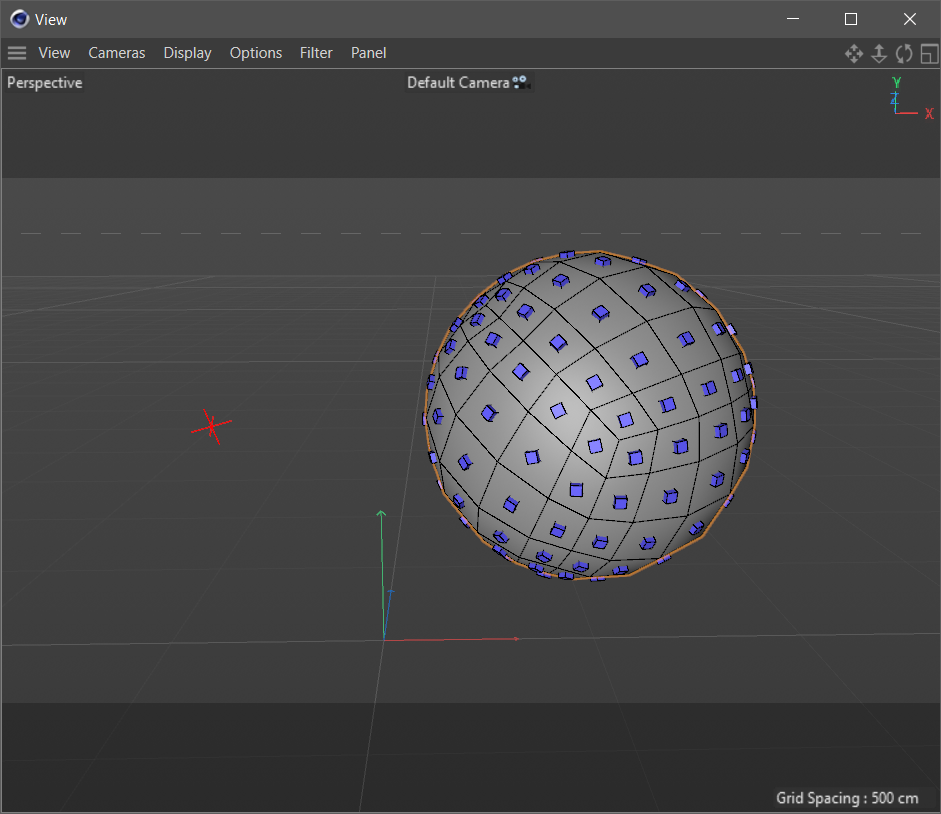The code:

``````"""Example for mimicking a Cloner Object in Object mode.

This is implemented as a Python effector but could also be transferred to
a custom ObjectData solution which does its own cloning. The solution
provided here leads to some problems when one has very irregular geometry,
for example, a landscape object in spherical mode with very high noise
settings and a very low tessellation. I.e., geometry that contains polygons
that are strongly non-co-planar. We cannot share code for how Cloner Object
solves this. It would be up to you to make this more robust.

As discussed in:
https://plugincafe.maxon.net/topic/13522/

References:
 Python Matrix Manual: https://developers.maxon.net/docs/
Cinema4DPythonSDK/html/manuals/data_algorithms/classic_api/matrix.html
"""

import c4d

def GetPolygonNormal(cpoly, points):
"""Returns the normal of a polygon.

Not needed in C++ due to CalcFaceNormal().
"""
if cpoly.c == cpoly.d:
return ~((points[cpoly.b] - points[cpoly.a]) %
(points[cpoly.c] - points[cpoly.a]))
else:
return ~((points[cpoly.b] - points[cpoly.d]) %
(points[cpoly.c] - points[cpoly.a]))

def GetPolygonSurfaceCenterPoint(cpoly, points):
"""Returns the surface center point for a polygon.

For a triangle, the arithmetic mean of all points will be returned. For a
quad, the midpoint of the edge dividing the triangle planes will be
returned. I.e., it will return the correct "center-point" for quads that
are not co-planar.
"""
if cpoly.c == cpoly.d:
return sum([points[cpoly.a], points[cpoly.b], points[cpoly.c]]) / 3.
else:
return (points[cpoly.a] + points[cpoly.c]) * .5

def GetMatrixFromNormal(normal, offset, upVector=None, epsilon=1E-5):
"""Constructs a transform for a given normal, offset and up-vector.
"""
# I am not going to explain this, since it is already being explained by
# the Python Matrix Manual .
up = c4d.Vector(0, 1, 0) if upVector is None else upVector
up = up if 1. - abs(normal * up) > epsilon else c4d.Vector(epsilon, 1, 0)

z = ~normal
temp = up % z
y = ~(z % temp)
x = ~(y % z)

return c4d.Matrix(off=offset, v1=x, v2=y, v3=z)

def main() :
"""Sets matrices of the clones in a fashion similar to the Cloner Object.
"""
# Get the Mograph particle data, the polygon object and the cloner object.
moData = c4d.modules.mograph.GeGetMoData(op)
polyObject = op[c4d.ID_USERDATA, 1]
clonerObject = op[c4d.ID_USERDATA, 2]

# Get out when we are missing data.
if (moData is None or
not isinstance(clonerObject, c4d.BaseObject) or
not isinstance(polyObject, c4d.PolygonObject)):
return False

# Get the total particle count and the particle matrices. And I am going
# to ignore fields for this effector.
particleCount = moData.GetCount()
particleMatrices = moData.GetArray(c4d.MODATA_MATRIX)

# Some polygon object data.
points = polyObject.GetAllPoints()
polygons = polyObject.GetAllPolygons()
polyCount = polyObject.GetPolygonCount()

# The transforms both for the cloner and the polygon object.
mgCloner = clonerObject.GetMg()
mgPoly = polyObject.GetMg()

# Check if either the polygon or clone count is larger. This is just me
# being lazy and not wanting to adjust the particle count of the cloner
# so that it matches the polygon count.
count = polyCount if polyCount < particleCount else particleCount

for i in range(count):
# Get the center point and normal for the polygon.
offset = GetPolygonSurfaceCenterPoint(polygons[i], points)
normal = GetPolygonNormal(polygons[i], points)

# Add the transform of the polygon object and subtract the transform
# of the cloner object from our point and normal, so that the clones
# will always stick to the polygon object.

# It is important that we include translations for the offset by
# using * (or Vector.Mul) and exclude them for the normals using ^
# (or Vector.MulV). Since the normals live in object space, including
# translations will mess up the orientation they represent.
offset = offset * mgPoly * ~mgCloner
normal = normal ^ mgPoly ^ ~mgCloner
# We also have to rotate our up-vector because otherwise the clones
# will rotate along that up-vector axis once the polygon object matrix
# is not the world frame.
upVector = c4d.Vector(0, 1, 0) ^ mgPoly ^ ~mgCloner

# Calculate and set the final particle matrix.
particleMatrices[i] = GetMatrixFromNormal(normal, offset, upVector)

moData.SetArray(c4d.MODATA_MATRIX, particleMatrices, False)
return True
``````

@ferdinand

Thanks for the response Ferdinand.

I'm sorry about the post, I'll make sure to follow the proper guidelines next time.

Your code worked great, problem successfully resolved.

John Terenece

Hello @JohnTerenece,

no worries, and great to hear!

Cheers,
Ferdinand

I am having an issue I believe is related to me running the code to generate the matrix that I am then feeding into the MoData. Shown with the picture below.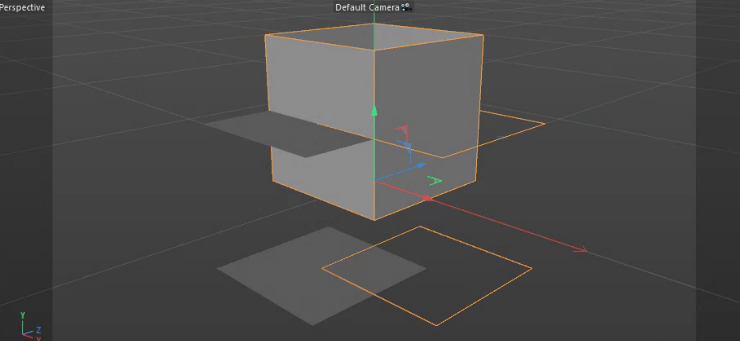Screenshot from deleted posting [added by @ferdinand for clarity]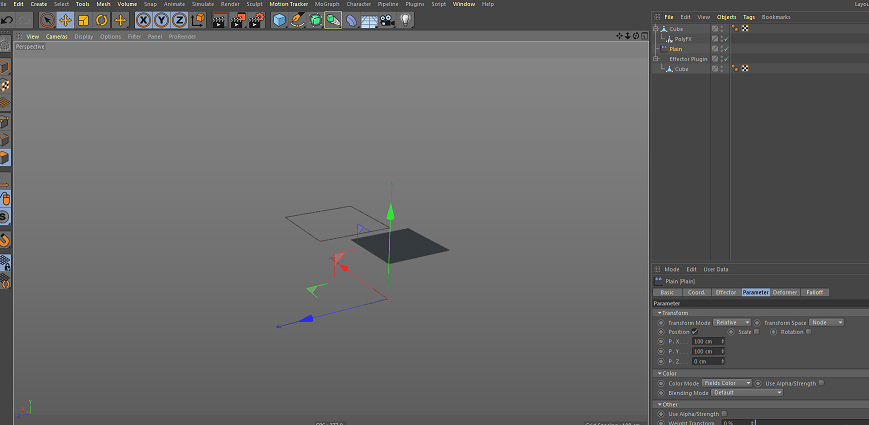The setup for my file is simple with two separate Cubes, one of them being used by my plugin the other being used by a PolyFX. Both my plugin and the PolyFX are being given the same Plain Effector.

If my code was working the way it's meant to the splines I'm creating would be matching the polygons of the PolyFX cube.

Here is the entirety of the function that I am using to get the points from the polygons and running the MoData code.

``````
maxon::BaseArray< maxon::BaseArray<Vector> > EffectPolygonClass::RunEffectors(BaseObject *op, BaseDocument *doc)
{

maxon::BaseArray<Vector> originalCenterPoint;
maxon::BaseArray< maxon::BaseArray<Vector> > finalConnections;
PolygonObject *poly = (PolygonObject*)doc->SearchObject("Cube"_s);

if (poly == nullptr)
return finalConnections;

Matrix polygonMatrix = poly->GetMg();

const CPolygon *polyArray = poly->GetPolygonR();

if (polyArray == nullptr)
return finalConnections;

Matrix objMx = poly->GetMg();
BaseObject *obj = (BaseObject*)Get();
BaseTag * motag = obj->GetTag(ID_MOTAGDATA);
if (motag == nullptr)
motag = obj->MakeTag(ID_MOTAGDATA);
if (motag == nullptr)
return finalConnections;
BaseTag * polyObjMocacheTag = obj->GetTag(ID_MOBAKETAG);

GetMoDataMessage modataMsg = GetMoDataMessage();
modataMsg.index = 0;

if (polyObjMocacheTag)
{
polyObjMocacheTag->Message(MSG_GET_MODATA, &modataMsg);
}
BaseTag *objTag = obj->GetTag(ID_MOTAGDATA);
if (objTag)
objTag->Message(MSG_GET_MODATA, &modataMsg);

if (!modataMsg.modata)
{
if (!motag->Message(MSG_GET_MODATA, &modataMsg) || !modataMsg.modata)
return finalConnections;
}

MoData *md = modataMsg.modata;
if (md)
{

maxon::BaseArray<Vector> centerPointArray;

maxon::BaseArray< maxon::BaseArray<Vector> > polyPointArray;
maxon::BaseArray<Vector> singlePointArray;
maxon::BaseArray<Vector> originalCenterArray;
md->GetAutoLock();
MDArray<Matrix> matrixArray;

Int32 polygonCount = poly->GetPolygonCount();
Int32 pointCount = poly->GetPointCount();
const CPolygon* polygons = poly->GetPolygonR();
const Vector *posArray = poly->GetPointR();

Matrix opMatrix = op->GetMg();
Matrix polyMatrix = poly->GetMg();
for (Int32 polyIndex = 0; polyIndex < polygonCount; polyIndex++)
{

Vector centerPointOfPolygon = Vector(0);
if (polygons[polyIndex].c == polygons[polyIndex].d)
{
resultVector = singlePointArray.Append(posArray[polygons[polyIndex].a]);
resultVector = singlePointArray.Append(posArray[polygons[polyIndex].b]);
resultVector = singlePointArray.Append(posArray[polygons[polyIndex].c]);

centerPointOfPolygon += posArray[polygons[polyIndex].a];
centerPointOfPolygon += posArray[polygons[polyIndex].b];
centerPointOfPolygon += posArray[polygons[polyIndex].c];
centerPointOfPolygon = (centerPointOfPolygon / 3.0);
}
else
{
resultVector = singlePointArray.Append(posArray[polygons[polyIndex].a]);
resultVector = singlePointArray.Append(posArray[polygons[polyIndex].b]);
resultVector = singlePointArray.Append(posArray[polygons[polyIndex].c]);
resultVector = singlePointArray.Append(posArray[polygons[polyIndex].d]);

centerPointOfPolygon += posArray[polygons[polyIndex].a];
centerPointOfPolygon += posArray[polygons[polyIndex].c];
centerPointOfPolygon = (centerPointOfPolygon / 2.0);
}
resultVector2D = polyPointArray.Append(singlePointArray);
singlePointArray.Reset();
resultVector = originalCenterPoint.Append(centerPointOfPolygon);
resultVector = centerPointArray.Append(centerPointOfPolygon);
}
md->SetCount(centerPointArray.GetCount());
matrixArray = md->GetMatrixArray(MODATA_MATRIX);

pointCount = poly->GetPointCount();
polygonCount = poly->GetPolygonCount();

BaseContainer *dataInstance = op->GetDataInstance();
const CustomDataType * effectorsraw = dataInstance->GetCustomDataType(ID_MG_MOTIONGENERATOR_EFFECTORLIST, CUSTOMDATATYPE_INEXCLUDE_LIST);
InExcludeData * effectors = nullptr;
if (effectorsraw)
effectors = (InExcludeData*)effectorsraw;

for (Int32 centerIndex = 0; centerIndex < centerPointArray.GetCount(); centerIndex++)
{
Vector normal = CalcFaceNormal(posArray, polyArray[centerIndex]);

Vector pointPos = polyMatrix * ~opMatrix * centerPointArray[centerIndex];
normal = polyMatrix * ~opMatrix * normal;
normal.Normalize();
Vector upVector = polyMatrix * ~opMatrix * Vector(0, 1, 0);
if (1.0 - abs(Dot(normal, upVector)) < 1E-5)
{
upVector = Vector(1E-5, 1, 0);
}
Vector z = normal;
z.Normalize();
Vector temp = Cross(upVector, z);

Vector y = Cross(z, temp);
y.Normalize();
Vector x = Cross(y, z);
x.Normalize();

matrixArray[centerIndex] = Matrix(pointPos, x, y, z);

}

if (effectors)
{
Effector_PassData emsg = Effector_PassData();
emsg.op = op;
emsg.md = md;
emsg.weight = 1.0;
for (Int i = 0; i < effectors->GetObjectCount(); i++)
{
BaseObject * e = (BaseObject*)effectors->ObjectFromIndex(doc, i);
if (!e)
continue;
if (!e->GetDeformMode())
continue;
e->Message(MSG_EXECUTE_EFFECTOR, &emsg);
}
GetMoDataMessage modataMatrixMsg;

}
MDArray<Matrix> itemsMtxArray = md->GetMatrixArray(MODATA_MATRIX);
Int32 matrixItemCnt = md->GetCount();

maxon::BaseArray<Vector> singleLevelReturn;

for (Int32 centerIndex = 0; centerIndex < matrixItemCnt; centerIndex++)
{
Matrix effectorMat = itemsMtxArray[centerIndex];

Vector savedPos = effectorMat.off;
Float distance = Distance(originalCenterPoint[centerIndex], savedPos);

Vector normalAngle = savedPos - originalCenterPoint[centerIndex];
normalAngle.Normalize();
for (Int32 pointIndex = 0; pointIndex < polyPointArray[centerIndex].GetCount(); pointIndex++)
{
resultVector = singleLevelReturn.Append((normalAngle * distance + polyPointArray[centerIndex][pointIndex]));

}
resultVector2D = finalConnections.Append(singleLevelReturn);
singleLevelReturn.Reset();
}
return finalConnections;
}

}

// Code used to create a placeholder Effector list
cid = DescLevel(ID_EFFECTORLIST, CUSTOMDATATYPE_INEXCLUDE_LIST, 0);
if (!singleid || cid.IsPartOf(*singleid, NULL))
{
BaseContainer bc;
bc = GetCustomDataTypeDefault(CUSTOMGUI_INEXCLUDE_LIST);

bc.SetString(DESC_NAME, "Effectors"_s);

bc.SetBool(IN_EXCLUDE_FLAG_SEND_SELCHANGE_MSG, true);

bc.SetInt32(IN_EXCLUDE_FLAG_BIG_MODE_SIZE, 150);
if (!description->SetParameter(cid, bc, DescMainTabGroup))
return TRUE;
}

}
``````

I went through the Python code and tried to follow it as closely as I could to generate the array of Matrixs that I use. This code matches the behavior of the PolyFX when using a Sphere but differs in the case of the Cube example.

I'm not entirely sure if the issue I'm having is a result of me not creating the Matrix properly or not utilizing the results from the MoData correctly but the issue is preventing me from moving on to trying to utilize rotation on Effectors.

John Terenece

Hello @JohnTerenece,

the PolyFX object is a deformer and modifies the deform cache of an object with the effectors that affect it. Your setup is not 100% clear to me, but I assume it looks like the one in your deleted posting.

Deformers like the Bend or PolyFX object generate a deform cache for an object. It can be retrieved with `BaseObject::GetDeformCache()`. Your code does not retrieve the deform cache for the "Cube" object. This means you operate on the geometry of "Cube" before deformation. You could fix it like this:

``````BaseObject* deformCache = op->GetDeformCache();
if (deformCache != nullptr)
poly = (PolygonObject*)deformCache;

Int32 polygonCount = poly->GetPolygonCount();
Int32 pointCount = poly->GetPointCount();
const CPolygon* polygons = poly->GetPolygonR();
const Vector *posArray = poly->GetPointR();
``````

This should fix your problem from what I can see here. However, there is the problem that this approach will not work when "Cube" is not a `PolygonObject` and instead a generator object that generates a polygon object, e.g., a Cube primitive. In this case the deform cache of the Cube primitive will be buried within the cache of the Cube primitive. The topic has been discussed in the past in the threads SceneHook and object caches and Unusual "NoneType" Error for GetDeformCache() among others.

Cheers,
Ferdinand

Thanks for the response Ferdinand.

I messed up the scene file in my post which is why I deleted it. The setup for my scene is this.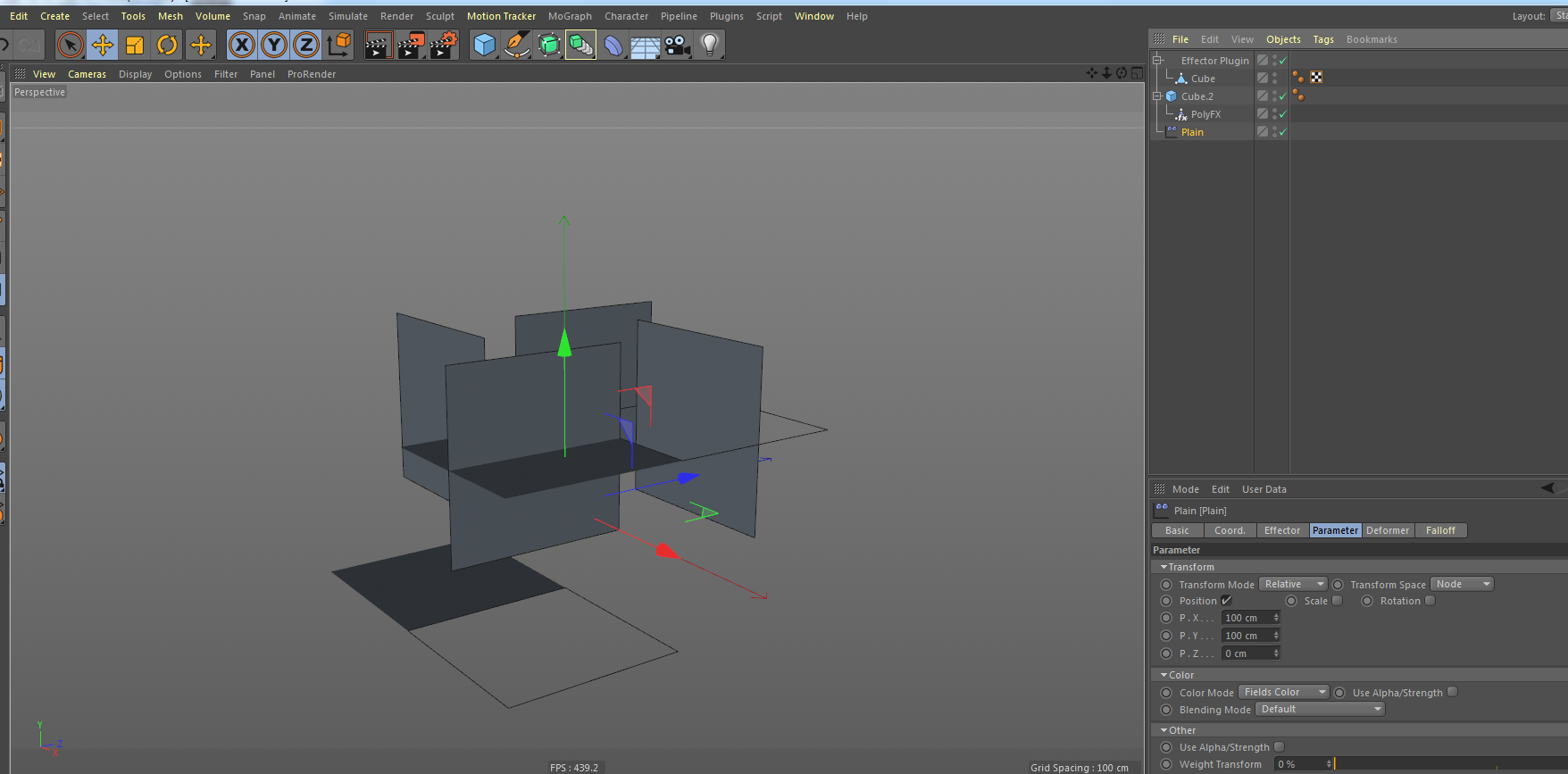Both my plugin and the PolyFX are being fed the Plain effector. I am trying to have my plugin take in polygons from a fed in object and then use the results from the effectors in the plugin to move those polygons to mimic the behavior of the PolyFX.

John Terenece

Hello @johnterenece,

so, to clarify this for myself. You have an `ObjectData` implementation which probably overwrites `GetContour`, i.e., builds a `SplineObject` , or draws spline-like information into the viewport. This plugin then has an `InExcludeData` parameter which is populated with Mograph effectors which are then meant to influence the vertices of your plugin. Consequently you want to do the deformation of your input geometry yourself and not like I assumed before not just clone stuff onto a `PolygonObject` that has been deformed by a `PolyFx`.

First of all, I do not see really the point in reinventing the wheel in the first place, why not use `PolyFX` if it does what you need anyways? I would also stress that this cobling up of functionalties, deformation and generation in your case, in a single plugin and even single function in your case, is often not a very good idea, bot for technical and debugging reasons.

As I said before, we cannot debug your code for you, but here are some points:

• The way you use a `MoData` tag is not its intended usage, the tag is reserved for MoGraph generators only and you hijacking it here is something you have to do on your own responsiblity.
• You also do not take the transforms of the effectors into account which might be a cause for your problem.
• There are other problems like using `BaseObject::MakeTag` and a possibly thread environment and the lack of error handling with `maxon::BaseArray::Append` which can lead to crashes, as you just store posible erros in `resultVector` and `resultVector2D` in your code which are undefined, but I assume to be of type `maxon::Result<Vector>` and `maxon::Result<maxon:BaseArray<Vector>`. But they are not the source of your problem, which likely lies in the fact that you do hijack `MoData` in the way you do and then do not respect the transforms of the effectors.

You still have not told us the plugin type you are implementing, but if it is a `ObjectData` which generates a spline, I would simply build the spline on the orginal polygon geometry and then retun the spline with a PolyFX attached to it in `GetContour`. Much easier than reinventing the wheel here. You would have to pass through the content of the `InExcludeData` of your plugin to the `PolyFX`.

Cheers,
Ferdinand